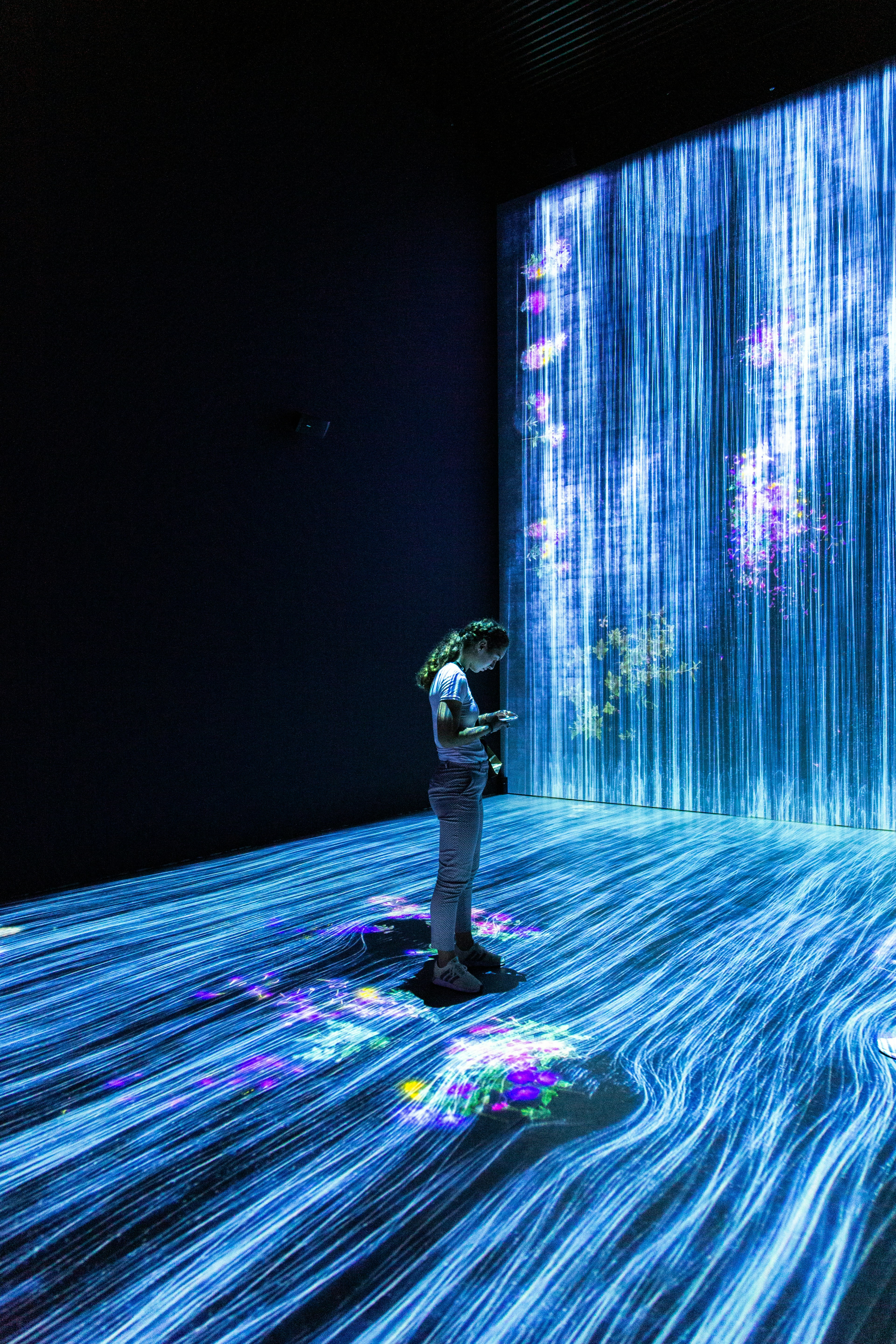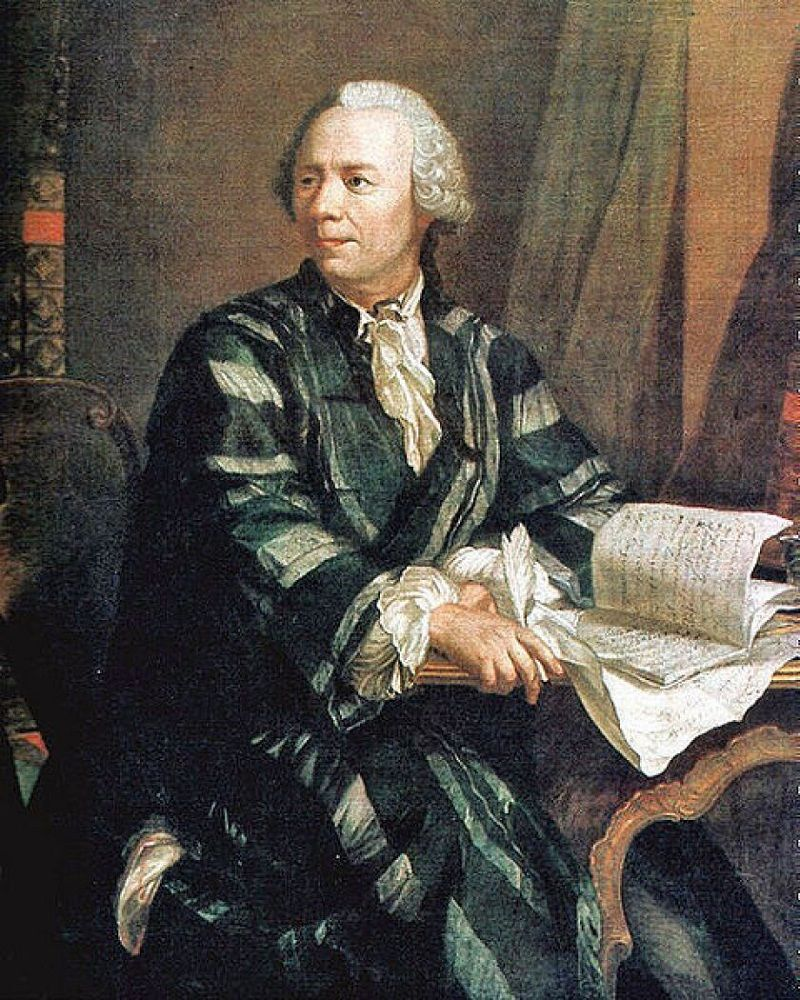2 Brilliant Breakthroughs in Computational Topology Using Machine LearningData scientist

Topology is a classical branch of mathematics, born essentially from Euler’s studies in the XVII century. It deals with the abstract notion of shape and geometry. The last decades were characterized by a renewed interest in topology and topology-based tools, due to the birth of computational topology and Spatial Data Analysis (SDA). Successful applications of computational topology to data analysis boosted a renewed interest in that field, and Spatial data analysis (SDA) has earned a prominent place in contemporary research, as a rich family of algorithms and methods from computational topology, e.g. Morse theory or persistent homology, to analyze and visualize data. Focusing on image analysis, in low dimensions (typically 2 or 3), techniques from SDA are used to extract and classify geometric features, e.g. level sets or integral lines. In this survey, I focus specifically on persistent homology, because I found this technique really promising with respect to the interplay with Machine Learning/Deep Learning.

# Table of content:

• Introduction - Basic introduction about computational topology
• Persistent Homology history - Discussing the history of persistent homology
• Evaluating basic notions of persistent homology
• Recent trends of computational homology with machine learning and neural networks
• Topological Layers
• Image and Shape processing
• Signal and time series analysis

# Persistent homology: history and basic notions

Algebraic topology is a branch of mathematics using tools from abstract algebra to study and characterize topological spaces. The basic aim is to find an algebraic invariant able to classify topological spaces up to homeomorphism. Persistent Homology bridges algebraic topology with the Morse theory core idea: exploring topological attributes of an object in an evolutionary context.

# Evaluating basic notions of persistent homologyIn order to understand the core idea of persistent homology, it is necessary to be familiar with the basics of algebraic topology. A simplicial complex is the standard algebraic object used to represent shapes of any dimension; simplices are its building blocks.

Def. A k-simplex is the k-dimensional convex hull of k+1 vertices. The convex hull of any nonempty subset of the k+1 vertices is called a face of the simplex.

A simplicial complex K is a set built from 0-dimensional simplices (0-simplices or points), 1- dimensional simplices (line segments), 2-simplices (triangles), 3-simplices (tetrahedra), and so on. The dimension of K is defined as the largest dimension of any simplex in it. To be a simplicial complex, K should satisfy the following conditions.

Def. A simplicial complex K is a set of simplices such that: - Every face of a simplex is also a simplex of K; - The intersection of any two simplices 1 and 2 in K is either a face of both 1 and 2 or the empty set.These conditions allow defining the boundary operator, which is fundamental to define the homology, an algebraic object computable (via linear algebra) for K that accounts for the number of connected components, holes, voids, etc. The boundary of a k-simplex is the formal sum of the (k-1)-dimensional faces. For example, the boundary of a triangle {,} of vertices, and is given by the sum of the edges More formally

({0,… , }) = ∑(k^i0 −1) {0, … , ^, … Vk} )

## HistoryFrosini, Ferri and collaborators

In a family of papers published(Above) between 1990 and 1993, Introduced the size functions, which are equivalent to the 0-dimensional persistent homology. The size functions are defined as functions from the real plane to the natural numbers which describe the shape of the objects (seen as sub-manifolds of Euclidean space). Also, different techniques of computation of size functions are provided, together with the definition of a deformation distance between manifolds measuring the difference in the shape of two manifolds, and applications to shape analysis.

In 2002 Edelsbrunner et al. Formalize the notion of persistence within the framework of filtration, which is the history of a growing complex. They introduced the classification of a topological event occurring during growth as either a feature or noise, depending on its lifespan within the filtration. The algorithm provided in this paper for computation yields only for subcomplexes of spheres and only with coefficients in F2.

# New Trends: Persistent homology In Machine Learning

The idea of allowing Machine Learning to learn topological information has been explored most frequently by feature engineering, looking at some predefined standard features conveying topological information. Persistent homology, as concerns data analysis, is a versatile method: there is no restriction to apply to any particular kind of data (such as images, sensor measurements, time-series, graphs, etc.). When we want to analyze an image, a shape, or a dataset generally

## Topological LayersIn the last five years(approx.), many research groups defined and implemented topological layers to exploit topological features in deep learning pipelines. Hofer et al. First developed a technique to input persistence diagrams into neural networks by introducing their own topological layer, able to learn a task-optimal representation during training. He proposes a differentiable Topology Layer that computes persistent homology, based on level-set filtrations and edge-based filtrations. It is publicly available and its implementation is based on PyTorch. A note: this layer may be placed at the beginning of a deep network and, using the fact that our input layer is differentiable, it can be used to perform adversarial attacks (gradient attack), i.e. cause a trained neural network to misclassify input.

PersLay is one of the first neural network layers, designed to handle persistence diagrams. It is based on a general framework for diagram vectorization: maybe the simplest way to generate a permutation-invariant and differentiable feature map is to turn each point of the persistence diagram into a vector, and then sum over all such vectors to eventually get a single vector. This is the core idea of Perslay: depending on the way the diagram points are turned into vectors and on the permutation-invariant operation that is being used, one can show that one can compute persistence images, persistence landscapes, and persistence silhouettes as particular instances of Perslay.

Very recently Kwangho Kim, Manzil Zaheer, and Joon Kim the authors propose PLLAY, a layer based on the weighted persistence landscapes. They show a tight stability bound that does not depend on the input complexity; therefore PLLAY is less prone to extreme topological distortions. Importantly, they provide guarantees of the differentiability of PLLAY with respect to the layer’s input: hence, such a layer may be placed anywhere in the network.

## Image and Shape Processing

The first applications of persistent homology were in 2D and 3D shape analysis, specifically in diverse tasks, i.e. classification, segmentation, retrieval, and many others. I list here just a few examples:

2006 A.Cerri & F.Cerri - the original size functions were used to generate 25 measuring functions for the automatic retrieval of trademark images, outperforming existing whole-image matching techniques;

2010 Skraba, P.Ovsjanikova - persistence-based clustering and the Heat Kernel Signature function are combined to achieve a multi-scale isometry invariant segmentation of deformable shapes.

Turner, K.Mukherjee - Demonstrated how persistent homology may be used to represent shapes and execute operations such as computing distances between shapes or classifying and modeling shapes and surfaces. Persistence descriptors have been used also statistical shape analysis.

## Signal processing and time series analysisEven if persistent homology originates in the context of image and shapes analysis, due to its versatility it was successfully and largely applied in signal processing and analysis. Indeed persistent homology provides efficient tools to denoise and analyzes both homogeneous and heterogeneous time series, and many researchers exploited topological features.

Perea and Harer used a sliding window approach to obtain a point cloud from a time series; the point cloud is then analyzed looking at periodicity as the repetition of patterns, quantifying this recurrence as the degree of circularity/roundness in the generated point cloud. This approach has applied data from gene expression and physiology, astronomical data, and weather.

Y. Umeda - proposed a novel approach for the classification of volatile time series: SDA is used to extract the structure of attractors, resulting in efficiency for both chaotic and non-chaotic time series, achieving performances improved of 18.5% compared to conventional approaches

The set of signals to which SDA can be applied is today quite rich; it includes, for example, physiological signals such as EEG/ECG and financial time series such as stock market indices. The analysis of market crashes in this Study is quite interesting because was the first application of SDA to this kind of data, providing a new type of econometric analysis, which complements the standard statistical measures, to perform a reliable early detection of early warning signals of imminent market crashes.

# References

1. Adams, H., Emerson, T., Kirby, M., Neville, R., Peterson, C., Shipman, P., Chepushtanova, S., Hanson, E., Motta, F., Ziegelmeier, L.: Persistence images: A stable vector representation of persistent homology. J. Mach. Learn. Res. 18(1), 218–252 (2017)
2. Barsocchi, P., Cassar´a, P., Giorgi, D., Moroni, D., Pascali, M.: Computational topology to monitor human occupancy. Proceedings 2(99) (2018)
3. Bauer, U., Kerber, M., Reininghaus, J.: Dipha (a distributed persistent homology algorithm). Software available at https://github.com/DIPHA/dipha (2014)
4. Bauer, U., Kerber, M., Reininghaus, J., Wagner, H.: Phat–persistent homology algorithms toolbox. Journal of symbolic computation 78, 76–90 (2017)
5. Biasotti, S., Cerri, A., Frosini, P., Giorgi, D. ad Landi, C.: Multidimensional size functions for shape comparison. Journal of Mathematical Imaging and Vision 32(2) (2008)